# Texas Go Math Grade 7 Lesson 14.1 Answer Key Personal Budgets

Refer to our Texas Go Math Grade 7 Answer Key Pdf to score good marks in the exams. Test yourself by practicing the problems from Texas Go Math Grade 7 Lesson 14.1 Answer Key Personal Budgets.

## Texas Go Math Grade 7 Lesson 14.1 Answer Key Personal Budgets

Texas Go Math Grade 7 Lesson 14.1 Explore Activity 1 Answer Key

Identifying the Components of a Personal Budget

It can be tough to reach a long-term goal without making a plan for how to get there. A budget is a plan to help you reach your financial goals.

Some of the components of a budget are income, or the amount of money you earn, and expenses, or how much you spend. Expenses can include planned savings for college, retirement, emergencies, and taxes.
The goal of a budget is to spend no more than you earn, and save some additional money if you can.

Jenna is saving for a tablet for college. She is making a 4-week budget. The budget shows her total income and the various expenses she has, including saving for the tablet.

Step 1
Enter the total amount that Jenna will earn babysitting for 4 weekends in the table.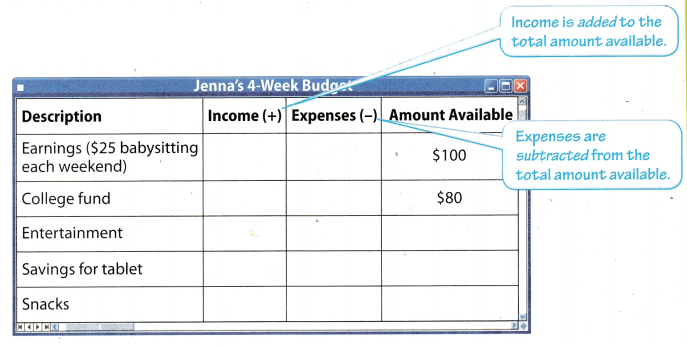Step 2
Enter Jenna’s expenses and planned savings in the Expenses column.
Expenses: entertainment—$15 snacks—$10
Planned savings: college fund—$20 tablet—$25
Step 3
Complete the Amount Available column by adding each row of income and subtracting each row of expenses.
How much money does Jenna have available at the end of 4 weeks? What could she do with it?

Reflect

Question 1.
What If? What if Jenna misses a weekend babysitting? How would that affect the budget?
Fixed expenses: guitar rental fee, saving for car, emergency saving.
Variable expenses: clothes, entertainment
Adding aLL the cost from the given table
Fixed expense = 15 + 30 + 10
= $55 Variable expense = 40 + 50 =$90

Identifying Types of Expenses

There are two different types of expenses: fixed expenses and variable expenses. Fixed expenses, such as car and house payments, occur regularly and do not change from month to month. A variable expense, such as purchases of food or gas, occurs regularly and is necessary for living, but you have some control over the amount.

Example 1.
A. The table shows Garrett’s monthly budget. To earn money, he mows lawns. Identify Garrett’s fixed and variable expenses.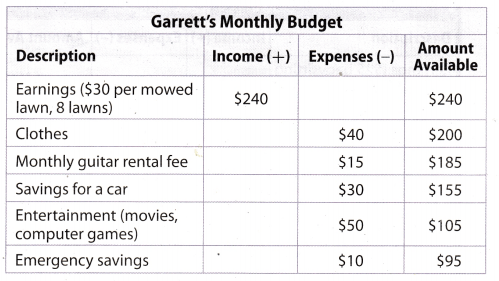Fixed expenses: guitar rental fee, savings for a car, emergency savings
Variable expenses: clothes, entertainment

B. The table shows Mrs. Everdeen’s monthly budget. Identify Mrs. Everdeen’s fixed and variable expenses.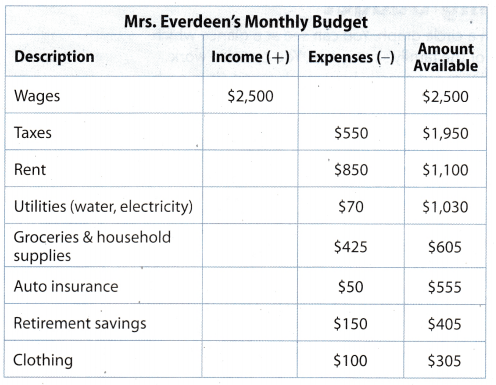Fixed expenses: taxes, retirement savings, rent, auto insurance Variable expenses: utilities, groceries and household supplies, clothing

Reflect

Question 2.
How are these budgets different?
Fixed expenses : taxes, retirenient savings, rent, auto insurance.
Variable expenses : utilities, groceries. clothing.
Adding all the cost from the given table
Fixed expense = 550 + 150 + 850 + 50
= $1600 Variable expense = 70 + 125 + loo =$595
In this budget $550 is given as taxes which is calculated as the fixed expenses, but the tax expense will depend upon tile amount wages or income which Mr. Everdeen gets each month. And the end of month he is left with$305 after all expenses.

Question 3.
Mallina is making a budget. The table shows the categories of expenses in her budget. In the second column of the table, use an F to mark fixed expenses and a V to mark variable expenses.snacks and entertainment are variable expenses.

Texas Go Math Grade 7 Lesson 14.1 Explore Activity 2 Answer Key

Analyzing a Family Budget

One way to present a budget is in a circle graph. You can see at a glance which categories take the greatest part of the family’s resources. You can also work backward from a circle graph to figure out exactly how much money is in each category.

Use the circle graph to complete the table for the Baker family’s monthly budget. Their net monthly income is $4,000.Step 1: Enter the income in the table. Step 2: Enter the percent or amount of money for each category from the circle graph in the table. Step 3: Calculate the amount of money or the percent for each category in the table. Step 4: Determine which expenses are fixed and which are variable. Place X’s in the appropriate columns. Step 5: Complete the Amount Available column.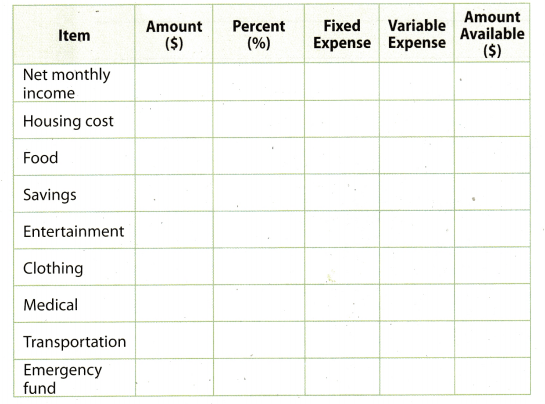Reflect Question 4. Analyze Relationships One month, the family must make an emergency car repair for$305. Are they able to pay for it out of the fixed emergency fund for that month? If not, how can they afford it?
Given net monthly income of Baker famiLy = $4, 000 Percentage of budget for emergency fund = 5% Cost of emergency car repair =$305
So, amount for emergency fund = $$\frac{5}{100}$$ × 4, 000
= 0.05 × 4000
= $200 The cost of emergency car repair is 305 but he has only$200 in his emergency fund. So he will not able to pay whole amount from emergency fund. Hence, he will need to take extra amount 305 – 200 = $105 from saving to pay the car repair cost. Question 5. The family wants to make a trip to Houston to visit the NASA Space Center. What are some ways they can save without using all of the allotted$160 for entertainment?
While on trip to Houston to visit NASA space centre. the ways in which family can save without using ail the allotted $160 for entertainment are reducing their variable expenses such as : 1. Food expenses 2. Clothing expenses YOUR TURN For 6-8, use the circle graph of the Torres family’s monthly budget. Their net monthly income is$4,500.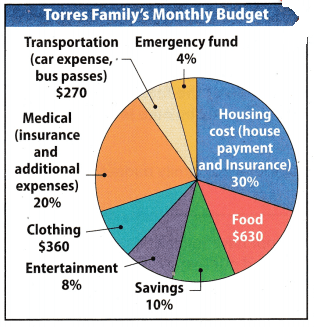Question 6.
How much does the Torres family Medical spend on housing each month?
Net monthly income of the Torres family = $4. 500 Percentage of budget a Lotted for housing = 30% Amount for housing = $$\frac{\text { Percent }}{100}$$ × Total amount Amount for housing = $$\frac{30}{100}$$ × 4500 = 0.3 × 4500 =$1350
Hence, the amount allotted each month for housing is 1350
Amount alloted each month for housing is 1350

Question 7.
What percent of the budget does the Torres family spend on food? clothing?
Net monthly income of the Torres famiLy = $4. 500 Amount spent on food each month =$630
Amount spent on clothing each month = $360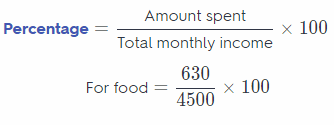= 0.14 × 100 = 14% For clothing = $$\frac{360}{4500}$$ × 100 = 0.08 × 100 = 8% Hence, 14% of budget is spent on food and 8% on clothing 14% of budget is spent on food and 8% on clothing Question 8. During a thunderstorm, lighting strikes a large tree in the Torres family’s backyard. The tree needs to be removed. The cost to remove the tree is$500. Is there enough money budgeted in the allotted emergency fund? Explain. If not, how much more does the family need, and how could they find the money for it?
Given net monthly income of Torres family = $4, 500 Percentage of budget for emergency fund = 4% Percentage of budget for saving fund = 10% Cost to remove tree from backyard =$500
So, amount for emergency fund = $$\frac{4}{100}$$ × 4, 500
= 0.04 × 4500
= $180 Amount for saving fund $$\frac{10}{100}$$ × 4. 500 = 0.1 × 4500 =$450

The cost of removing tree is 500 but he has only $180 in his emergency fund. So he will not able to pay whole amount from emergency fund. Hence, he will need to take extra amount 500 – 180 =$ 320 from saving to pay for removing tree from background.
Extra $320 is needed from saving to pay for removing tree Texas Go Math Grade 7 Lesson 14.1 Guided Practice Answer Key Sofia is creating a budget to save$250 for a week of soccer camp. She earns money by working as a mother’s helper on Saturday mornings and Wednesday afternoons. She earns $20 each time she works. She wants to save$12.50 a week for the camp. Her mother wants her to save $5 a week for college. She is planning on spending$5 a week for snacks and $10 a week for entertainment. Question 1. Complete the table. (Explore Activity 1)Answer:Total income of a month is$ 160

Question 2.
Circle the variable expenses in Sofia’s budget. (Example 1)
The Variable expenses of the Sofia’s 4-week budget are
Snacks = $20 Entertainment =$40
The Fixed expenses of the Sofia’s 4-week budget are:
Saving for soccer camp = $50 Saving for college =$20
Hence, total variabLe expenses is 20 + 40 = $60 Total variable expenses is$60

Question 3.
If Sofia decides to take piano lessons at the community center for $5 a week, would that be a fixed expense or a variable expense? ________ (Example 1) Answer: Fixed expense If Sofia decides to take Piano lessons at the community centre for the$5 then this cost will be regarded as the fixed expanse. Because every week Sofia has to pay $5 at community centre. And this cost will be fixed, which will not change once Sofia is enrolled for classes. So total Sofia has to pay$20 in a month.

For 4-5, use the circle graph of the Talbot family’s Talbot monthly budget. Their monthly income is $3,200. (Explore Activity 2)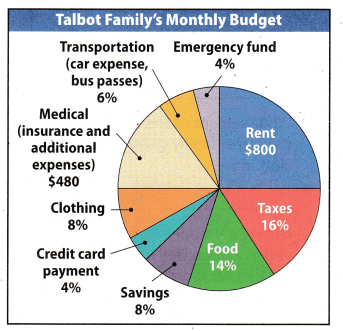Question 4. What percent of the Talbot family budget is 6 spent on rent? _______________________________ Answer: Net monthly income of the Talbot family =$3. 200
Amount of budget allotted for rent = $800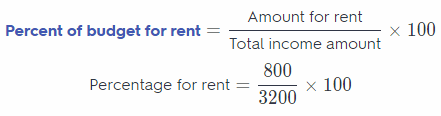= $$\frac{1}{4}$$ × 100 = 25% Mence, trie percentage of budget allotted each month for rent is 25% Percentage of budget allotted each month for rent is 25% Question 5. If the Talbots did not have to make a credit card payment, and instead put that money into savings, how much would they be able to save each month? _________________________ Answer: Net monthly income of the Talbot family =$3,200
Percentaget of budget allotted for credit card = 4%
Percentaget of budget allotted for saving = 8%
Amount of budget for credit card = $$\frac{\text { Percent }}{100}$$ × Total monthly income
Amount allotted for credit Savings = $$\frac{4}{100}$$ × 3, 200
= 0.04 × 3200
= $128 Amount alloted for savings = $$\frac{8}{100}$$ × 3,200 = 0.08 × 3200 =$ 256
Total saving in month = 128 + 256 = $384 Hence, the total saving of the month is$384
Total saving in a month is $384 Essential Question Check-in Question 6. How does a budget help an individual or a family manage their finances? Answer: Budget helps an individual or a family manage their finance in many ways such as when there is an emergency need of money, than in that tough situation we are able to manage that amount from the emergency fund of the budget. Also when there is something we want to buy in future like car, house, etc for that also we can fix a small percentage from our budget to achieve that. So simply budget makes us the worry free in month. Budget makes the individual or family burden free. Texas Go Math Grade 7 Lesson 14.1 Independent Practice Answer Key For 7-8, use the table.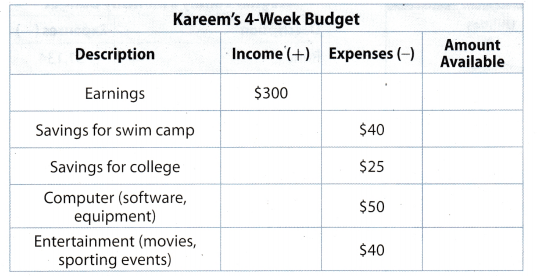Question 7. Complete the Amount Available column in the table. Answer: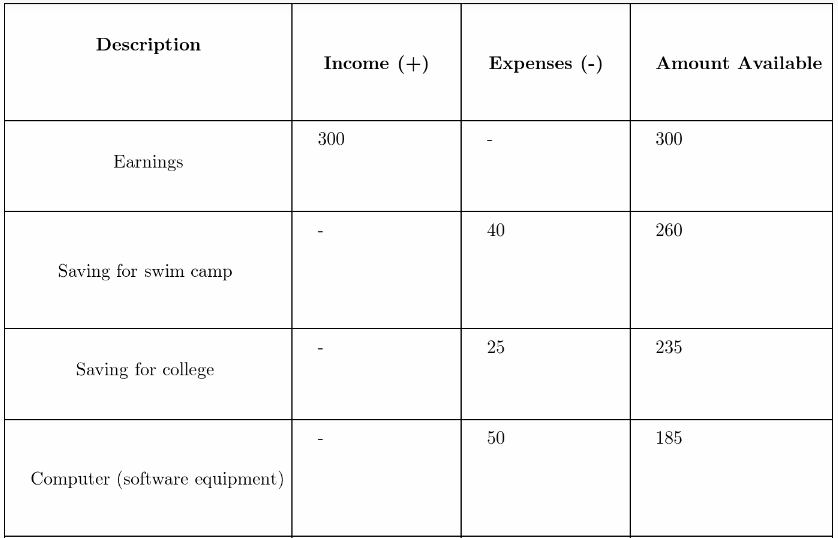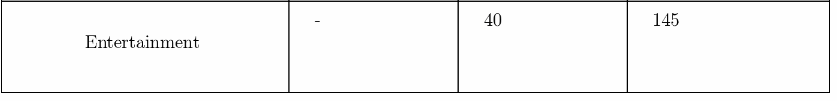saving after all expense is$145

Question 8.
The swim camp costs $280. How long will it take Kareem to save enough for the camp? Show your work. Answer: Given total cost of swim camp =$280
Saving of Kareem for swimming camp per month = $40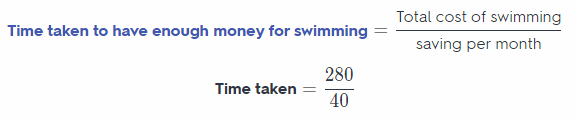= 7 Hence, it will take 7 months to save enough money for camp 7 months For 9-12, use the circle graph for the Moore family’s monthly budget. Their monthly income is$5,000.Question 9.
What percent of the Moore’s income goes to taxes?
Net monthLy income of the Moore’s family = $5, 000 Amount that goes for the taxes =$850= 0.17 × 100
= 17%
Hence, the percentage of income that goes to taxes is 17%
Percentage of income that goes to taxes is 17%

Question 10.
How much does the Moore family spend each year on insurance?
Net monthly income of the Moore family = $5, 000 Percentage of budget allotted for insurance = 12% Amount of budget for insurance = $$\frac{\text { Percent }}{100}$$ × Total monthly income Amount allotted for insurance each month = $$\frac{12}{100}$$ × 5,000 = 0.12 × 5000 =$600
Yearly spending of Moore famiLy on insurance = 12 × Monthly spending
Yearly spending on insurance = 12 × 600 = $7, 200 Question 11. Draw Conclusions Emily says that the Moore family spends$300 more on recreation than on transportation. Is she right? Explain.
Net monthly income of the Moore’s family = $5, 000 Amount that spent on recreation =$500
Percentage go budget spent on transportation = 6%
Amount of budget for transportation = $$\frac{\text { Percent }}{100}$$ × Total income amount
Amount for transportation = $$\frac{6}{100}$$ × 5,000
= 0.06 × 5,000
= $300 Difference in amount = Recreation – Transportation = 500 – 300 =$200
So, Emily is not correct because Moore’s family spend $200 more on recreation than on transportation not$300 as
Hence, the percentage of income that goes to taxes is 17%
No, Emily is not correct.

Question 12.
Communicate Mathematical Ideas. The Moore’s monthly income increases by $500, but the mortgage expense stays the same. Explain how to find what percent of the budget the mortgage payment would now be. Answer: Initially monthly income of Moore’s family =$5, 000
Amount of increase in monthly income = $500 Percentage of budget for mortgage = 22% It is given in problem that the mortgage expense remains same even after their is increase of$500 in monthly income
Amount of mortgage expense = $$\frac{22}{100}$$ × 5,000
= 0.22 × 5,000
= $1100 New monthly income of Moore’s family 5000 + 500 =$5, 500
New percentage mortgage expense = $$\frac{1100}{5,500}$$ × 100
= $$\frac{100}{5}$$
= 20%
Hence, new percentage of mortgage expense is 20%
New percentage of mortgage expense is 20%

H.O.T. Focus on Higher Order Thinking

The circle graph shows the Rivera family’s monthly budget. Their monthly income is $4,500. The table shows the budget for the Wagner family. Their monthly income is$4,200.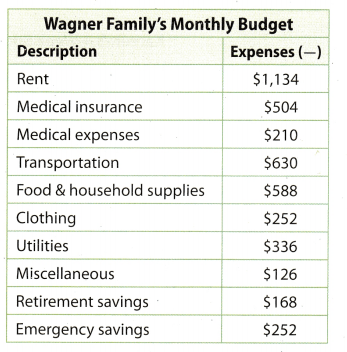Question 13.
Analyze Relationships Which family pays a greater part of their income for transportation (including car payments)? Explain your conclusion.
Monthly income of the Rivera family = $4. 500 Percentage of budget for transportation = 8% Percentage of budget for Car payment = 4% Amount spent on transportation = $$\frac{8}{100}$$ × 4, 500 = 0.08 × 4.500 =$360
Amount spent on Car payment = $$\frac{4}{100}$$ × 4, 500
= 0.04 × 4.500
= $180 Total Money spent by Rivera family on transportation = 360 + 180 =$540
Total Money spent by Wagner family on transportation = $630 Hence, Wagner famiLy spend more on transportation as compare to the Rivera family. Wagner family spend more on transportation as compare to the Rivera family. Question 14. Multistep Over a year, which family pays more for medical insurance? How much more? Answer: Monthly income of the Rivera family =$4, 500
Percentage of budget medical insurance = 10%
Amount spent on Medical insurance = $$\frac{10}{100}$$ × 4, 500
= 0.1 × 4,500
= $450 Rivera family spending on Medical insurance in year = 12 × 450 =$5. 400
Wagner famiLy spending on medical insurance in year = 12 × 504 = $6,048 Difference of medical insurance spending =$6,048 – $5. 400 =$648
Hence, Wagner family pays more for medical insurance in a year and the amount is $648 more as compared to the spending of Rivera family. Wagner family spend$648 more on medical insurance.

Question 15.
Multiple Representations Compare the two budgets. What are the advantages and disadvantages of each type of presentation?

• Pie chart represent! t lie large set of data in visual form.
• Pie chart shows the relative proportion of data.
• In pie chart circle is made in proportion of whole data.
• Pie chart does not reveals the exact values.
• If there are too many data then it becomes less effective.